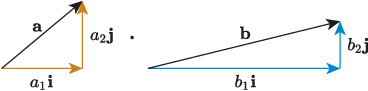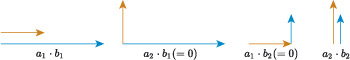Science, Maths & Technology

### Become an OU studentIntroducing vectors for engineering applications

Start this free course now. Just create an account and sign in. Enrol and complete the course for a free statement of participation or digital badge if available.

# 4.1 Scalar product of a vector from components

Consider vectors and in Figure 29. In component form these are written as and . How can we calculate the scalar product ?Figure 29 Finding the scalar product of and by comparing components

The scalar product will tell us how much vector will grow vector , and to determine this we want to identify how much the vectors interact. One method is to consider how much the horizontal and vertical components of the vectors interact, as illustrated in Figure 30. There are four possible combinations to consider: horizontal to horizontal, horizontal to vertical, vertical to horizontal, and vertical to vertical.Figure 30 Interacting component vectors in the scalar product of and

Horizontal components do not interact with vertical components (and vice versa) because they are independent of each other, so and , and they do not contribute to the value of scalar product. Horizontal components interact with each other, and vertical components interact with each other, so and both contribute to the value of .

The expression is a measure of how much the scalar quantity grows the scalar quantity , so it is equal to , and similarly is equal to . The scalar product is a combination of these, so

For example, if and , then

## Scalar product of vectors in terms of components

If and , or and in column notation, then

## Activity 19

Suppose that , and . Find the following.

• a.

• b.

• c.